# Regular Polygon - Definition with Examples

The Complete K-5 Math Learning Program Built for Your Child

• 40 Million Kids

Loved by kids and parent worldwide

• 50,000 Schools

Trusted by teachers across schools

• Comprehensive Curriculum

Aligned to Common Core

What is a Regular Polygon?

A 2-dimensional enclosed figure made by joining 3 or more straight lines is called a polygon. Polygons are also known as ‘flat figures’.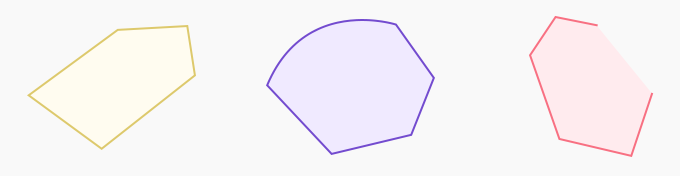Polygon                                    Not a Polygon                                Not a Polygon
(straight sides)                               (has a curve)                              (open, not closed)

A polygon having equal sides and equal angles is a regular polygon.

Parts of a regular polygon

A regular polygon has three parts:

• Vertices

• Angles: interior and exterior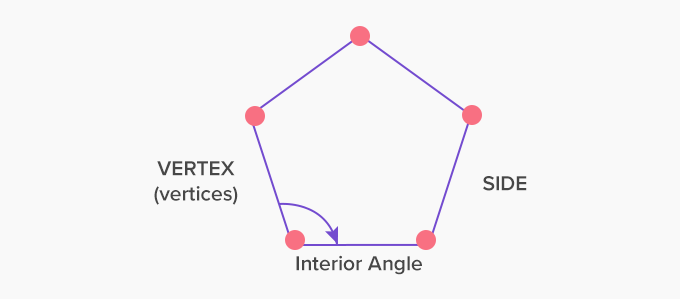The interior angle is the angle formed within the enclosed surface of the polygon by joining the sides.

Different regular polygons

The names of the regular polygons come from the number of sides they have.

 Name Figure Sides Interior Angle Equilateral triangle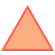3 equal sides Each angle is 60° Square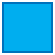4 equal sides Each angle is 90° Pentagon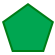5 equal sides Each angle is 108° Hexagon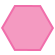6 equal sides Each angle is 120° Heptagon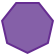7 equal sides Each angle is 128.57° Octagon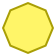8 equal sides Each angle is 135°

 Fun facts about polygon: A circle is a regular 2D shape but it is not a polygon because it does not have any straight sides. Each angle of a regular polygon can be calculated by the given formula: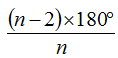where n is the number of sides in the regular polygon.

Won Numerous Awards & Honors